Overview

Working with MUMIE as author

Working with MUMIE as teacher

Go back to the old wiki

We revise and update this wiki. We apologize for the inconvenience this may cause you.

# Type of problem, question and answer

The content of every generic TeX problem is included in a problem environment which must be contained only once in TeX files.

The use of the \usepackage declaration is mandatory too. WebMiau creates it by using the code snippet creator.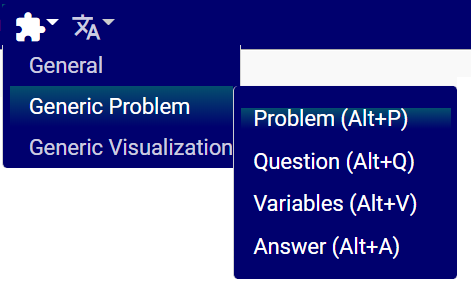Every question is defined in a question environment inside problem. The structure looks as following:

12345678910\usepackage{mumie.genericproblem}\begin{problem}    \begin{question} % start of question 1      ...    \end{question}     \begin{question} % start of question 2      ...    \end{question}\end{problem} 

Within the question environment, one must define a type using the \type{...} command.

\type{...} can be set for the whole group of questions, but can also be set individually for each question and answer.
This way, it is possible to create a question with have different types of answers.

The position of the command determines how it works.

123456789101112\begin{problem}  \type{...} % set for all questions   \begin{question}    \type{...} % or set only for this question and all their answers     \begin{answer}      \type{...} % or set only for this answer    \end{answer}             \end{question}         \end{problem} 
Notice that some features like \permuteAnswers won't be available if a question has different types of answers.

## What type is applicable

Type Preview
input.number

more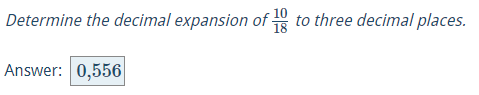input.finite-number-set

more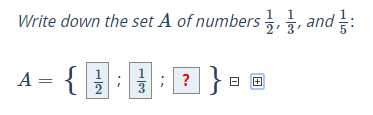input.function
with the pre-given solution

more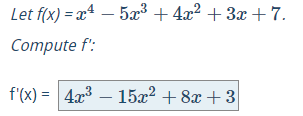input.interval

Details
input.cases.function
Designed for case differentiations of e.g. absolute value functions.
Note that you can only use this input type in an answer environment.

more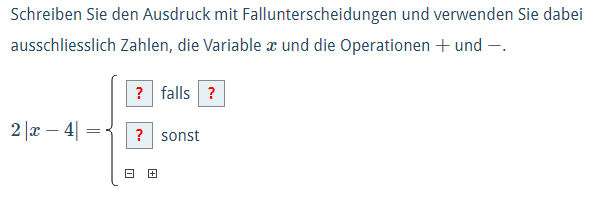input.matrix
If the solution to a question is a row vector, a column vector or a matrix,
the generic problem is of the type input.matrix.

example in WebMiau (cross product)

Use checkAsFunction for comparing matrices with (multivariate) functions as matrix entries.

example in WebMiau (Jacobian matrix)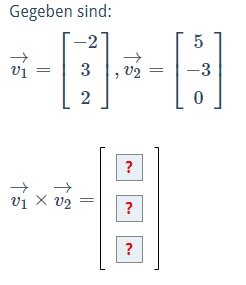input.text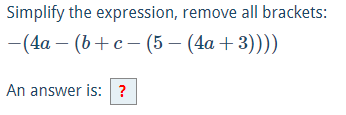input.truth-table

Details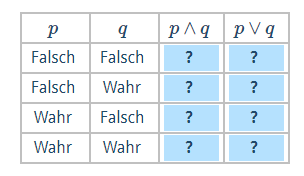mc.matrixmc.unique

moremc.yesno

moremc.multiple

more## Overview input field questions

More detailed information on input field questions can be found under the following link:
Overview input field questions

# Number fields

All variables and functions are interpreted in a number field which can be defined by the \field{...} command. It can take one of the following values:

number field Description
real real numbers (i.e. double values); this is the default value if the \field{number field} command is omitted
integer integer numbers
rational rational numbers
complex complex numbers with real numbers as real and imaginary parts
complex-rational complex numbers with rational numbers as real and imaginary parts
123456789101112\begin{problem}  \field{...} % set for all questions   \begin{question}    \field{...} % or set only for this question and all their answers     \begin{answer}      \field{...} % or set only for this answer    \end{answer}             \end{question}         \end{problem} 

# Variables

Questions can become dynamic and personalised through the use of variables and functions. These must be defined
in a variables environment

The environment \begin{variables} ... \end{variables} can be set for the whole group of questions, but can also be
set individually for each question. The position of the command determines how it works.

12345678910111213141516\begin{problem}     \begin{variables} % set for all questions      ...    \end{variables}     \begin{question}      \begin{variables} % set only for this questions        ...      \end{variables}       \begin{answer}      \end{answer}               \end{question}         \end{problem} 

## Example

1234567891011\begin{question}   \begin{variables}       \number{a}{12}       \number{b}{4}       \number{c}{10}       \number{d}{5}       \function{f}{a/b}       \function{g}{c/d}   \end{variables}   ...\end{question} 

Will result in variables

• $$a=12$$,
• $$b=4$$,
• $$c=10$$,
• $$d=5$$ and
• functions $$f=\frac{12}{4}$$ and $$g=\frac{10}{5}$$

## Commands

Command Description Details
\number A fixed number variable, this can be any kind of number. The field command determines how the number will be interpreted.
\function A function definition, the definition may include variables and other functions.
When an included variable is not defined, it will be a free variable.
Again, the field command determines how the numbers within the function will be interpreted.
function
\derivative Creates a new function representing the derivative of the expression/variable that was passed into it. derivative
\substitute Creates a new function that is the composition of two other functions. substitute
\randint A random integer number, including zero (same as randint[z])
\randint[Z] A random integer number, excluding zero Random numbers
\randdouble A random double number, including zero Random numbers
\randrat A random rational number, including zero Random numbers
\drawFromSet Randomly draw a number (or string) from a set Random numbers
\randadjustIf Random variables can be adjusted to satisfy a specific constraint. randadjustIf

## Using Variables in text

All variables can be used in texts (e.g. question task, answer text) by using the tex command \var with the name of the variable as argument:

12345678910111213\begin{question}   \begin{variables}    \function{f}{a/3}  \end{variables}   \text{Determine the decimal expansion of $\var{f}$ correct to three decimal places.}   \begin{choice}    \text{$\var{solution1}$}  \end{choice} \end{question} 

## Random numbers

Random numbers can be used instead of fixed numbers to personalize problem data.

Example

123456789\begin{question}   \begin{variables}      \randint[Z]{x1}{-10}{10}  %\randint[Z]{name}{min}{max}      \randdouble{x2}{0}{1}      \randrat{name}{minNumerator}{maxNumerator}{minDenominator}{maxDenominator}  \end{variables} \end{question} 

\drawFromSet Draw randomly a number (or string) from a set

• parameters \drawFromSet[options]{name}{set}
• numbers in the set can be integer, rational or double numbers
• separator in the set can be any (non white space) character, default separator is the comma
• type is either number or string, default type is number
• multiple options are separated by a space (see example below)
12345678910\begin{question}   \begin{variables}      \drawFromSet{a}{1,2,3,4,5,6}      \drawFromSet{b}{1/2,5,1.3,9/11,42}      \drawFromSet[separator=;]{c}{1;2.1;3.5;9}      \drawFromSet[separator=; type=string]{d}{hello;world}  \end{variables} \end{question} 

## Variable pools

Instead of using random values for numbers it is possible to define several sets of values inside a so called variable pool.

• A pool consists of several variables environments holding each the elements for a single set of variables.
12345678910111213141516171819\begin{question}   \begin{pool}    \begin{variables}      \randint{a}{1}{3}      \drawFromSet{b}{1,2,3}      \randadjustIf{a,b}{a = b}      \function[calculate]{f}{2*a+b}    \end{variables}         \begin{variables}      \randint{a}{-3}{-1}      \drawFromSet{b}{-1,-2,-3}      \randadjustIf{a,b}{a = b}      \function[calculate]{f}{2*a-b}    \end{variables}      \end{pool} \end{question} 
• A single set will be chosen randomly for every homework problem. The following example shows a pool with two sets of variables:
1234567891011121314151617\begin{question}   \begin{pool}       \begin{variables}          \number{a}{7}          \number{b}{3}      \end{variables}       \begin{variables}          \number{a}{5}          \number{b}{6}      \end{variables}   \end{pool} \end{question} 
Variable pools can only be used inside a question. There are no global variable pools at the moment.

# Compute solutions in MC questions

When using the compute option in multiple choice answers inside the \solution command an additional \iscorrect
command is required.

It constructs a relation and takes three arguments:

1. left side of the relation; must be a function variable
2. relation sign (i.e. <, >, =, >=, <=, !=)
3. right side of the relation; must be a function variable

When the relation solves to true then the correct answer for this choice will be yes:

123456789\begin{question}    ...    \begin{choice}        \text{$\var{g}$}        \solution{compute}        \iscorrect{g}{<}{f}    \end{choice}    ...\end{question} 

Complex Example

# Explanations

With \explanation the author can define a feedback that the user gets if an answer wasn't (completely) correct.
Usage: \explanation[optional condition]{text}.

More details

# Consecutive errors

The automatic correction of generic TeX problems can take into account consecutive errors. This feature is only
available for questions of type input.number or input.function.

Consider a problem with several questions, all of them of type input.number or all of them of type input.function.
Assume the solution of the n-th question depends on the solution of the m-th question for some question numbers $$m$$,$$n$$
with $$m < n$$. Say the student's solution of the m-th question was wrong. Then the student used a wrong input for the
n-th question. Assume that, apart from starting with a wrong input, the student solved the n-th question correctly.

This is what we call a consecutive error.

Taking into account consecutive errors means the student doesn't get the points for the m-th question, but the solution
of the n-th question is counted as correct and the points are awarded.

Taking into account consecutive errors works as follows. The questions are corrected one after the other.

For each question, the following happens:

1. First, the question is corrected normally, meaning the student's solution is compared to the correct solution.
2. If the student's solution coincide with the correct solution, everything is ok and the correction proceeds to the
next question.
3. Otherwise, the correction of this question is repeated with certain variables bound to student answers of previous
questions. This is called conditional correction. Which variable is bound to which answer is controlled by the author
using the \earlierAnswer command described below.
4. The score resulting from the conditional correction is compared to that of the normal correction. The correction
with the higher score counts.

The \earlierAnswer command has the following form:

\earlierAnswer{VARIABLE}{QUESTION_NUMBER,ANSWER_NUMBER}

where VARIABLE is the name of the variable defined on global (not question or answer) level, QUESTION_NUMBER
the number of the question and ANSWER_NUMBER the number of the answer.

If another variable depends on a variable that is referenced in \earlierAnswer, the dependent variable must also be defined in the global (and not in a question/answer) variable environment.

The answer number and the comma before it can be omitted:

\earlierAnswer{VARIABLE}{QUESTION_NUMBER}

In that case, the answer number defaults to 1.
Optionally you can set the QUESTION_NUMBER to -1 to refer to a previous answer in the same question. This is
needed when you for example use \randomquestionpool (see Randomquestionpool) at the begin of a problem to make a selection:

\earlierAnswer{VARIABLE}{-1,ANSWER_NUMBER}

The \earlierAnswer command is only allowed in the "variables" environment of a question.

Do not use the command \earlierAnswer to refer to an earlier question in a problem that has \randomquestionpool enabled! Problems with random question pools can only use \earlierAnswer for subtasks within the same question, by using the value -1 for the question number.

Below is a complete example of a problem. The problem is trivial: a random number Q is given, and the student has to
compute $$x = Q + 1$$ in the first question, $$y = x + 1$$ in the second, and $$z = y + 1$$ in the third. The second
and third question depend on the solutions of previous questions. To take this into account, we added \earlierAnswer
commands to bind variables to earlier answers. In the second question, for example, we bound x to the first answer of
question 1.

123456789101112131415161718192021222324252627282930313233343536373839404142434445464748495051525354555657585960616263646566\usepackage{mumie.genericproblem} \title{Scratch} \begin{problem}         \begin{variables}                \randint{Q}{-4}{4}                \function{x}{Q+1}                        \function{y}{x+1}                        \function{z}{y+1}                \end{variables}         \begin{question}                \text{\textbf{Step 1}\\ \textit{Let Q = $\var{Q}$. Compute x = Q + 1:}}                \explanation{Simply add one}                \type{input.number}                \precision{3}                \field{real}                 \begin{answer}                        \text{ x = }                        \solution{x}                \end{answer}        \end{question}         \begin{question}                 \begin{variables}                        \earlierAnswer{x}{1,1}                \end{variables}                 \text{\textbf{Step 2}\\ \textit{Compute y = x + 1:}}                \explanation{Simply add one}                \type{input.number}                \precision{3}                \field{real}                 \begin{answer}                        \text{ y = }                        \solution{y}                \end{answer}         \end{question}         \begin{question}                 \begin{variables}                        \earlierAnswer{x}{1,1}                        \earlierAnswer{y}{2,1}                \end{variables}                 \text{\textbf{Step 3}\\ \textit{Compute z = y + 1:}}                \explanation{Simply add one}                \type{input.number}                \precision{3}                \field{real}                 \begin{answer}                        \text{ z = }                        \solution{z}                \end{answer}         \end{question} \end{problem} 

# Random question pools

With the command \randomquestionpool, one can tell Mumie to randomly choose one question from a set of questions within a problem.
Usage: \randomquestionpool{number of first pool-question}{number of last pool-question}

Take care that problems with random question pools can only use \earlierAnswer
for subtasks within the same question (see above).

More details